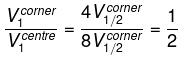Courses

# Test: Electrostatic Potential

## 20 Questions MCQ Test Physics Class 12 | Test: Electrostatic Potential

Description
This mock test of Test: Electrostatic Potential for JEE helps you for every JEE entrance exam. This contains 20 Multiple Choice Questions for JEE Test: Electrostatic Potential (mcq) to study with solutions a complete question bank. The solved questions answers in this Test: Electrostatic Potential quiz give you a good mix of easy questions and tough questions. JEE students definitely take this Test: Electrostatic Potential exercise for a better result in the exam. You can find other Test: Electrostatic Potential extra questions, long questions & short questions for JEE on EduRev as well by searching above.
QUESTION: 1

### The minimum velocity v with which charge q should be projected so that it manages to reach the centre of the ring starting from the position shown in figure is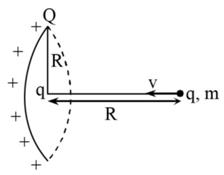Solution: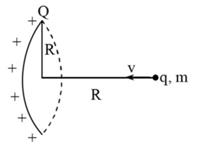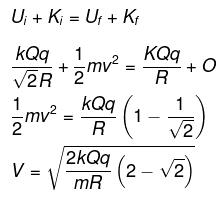QUESTION: 2

Solution:

Definition based

QUESTION: 3

### Electric field intensity is equal to

Solution:

Definition based

QUESTION: 4

Electric potential at a point located far away from the charge is taken to be

Solution:

Electric potential at a point located far away from the charge is taken to be zero Because the distance is much larger so in other words there is no effect of other charges on that charge.

QUESTION: 5

A charge of 6 mC is located at the origin. The work done in taking a small charge of -2 x 10-9 C from a point P (0, 3 cm, 0) to a Q (0,4 cm, 0) is​

Solution:

q=6x10-10 c
Q=-2x10-9c
r1=3x10-2m
r2=4x10-2m
△vQ=w/q
(1/4πεo)-2x10-9/10-2[(1/4)-(1/3)]=W/6x10-3
9x109x2x10-9x6x10-3/12x10-2=W
W=9x10-3x102
W=0.9J

QUESTION: 6

A particle of mass 1 Kg and charge 1/3 μC is projected towards a non conducting fixed spherical shell having the same charge uniformly distributed on its surface. The minimum intial velocity V0 of projection of particle required if the particle just grazes the shell is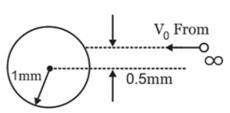Solution:

From conservation of angular momentum,
mr0 = r/2 = mvr
⇒ v = v0/2
From conservation of energy,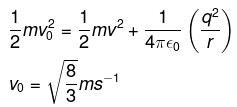QUESTION: 7

On moving a charge of 20 coulombs by 2 cm, 2 J of work is done, then the potential difference between the points is

Solution:

Potential difference between two points is given by

Va - Vb = W/q0

Work, W = 2 J

Charge, q0 = 20 C

Potential difference = 2/20 = 0.1 V

The correct option is C.

QUESTION: 8

The amount of work done in moving a charge from one point to another along an equipotential line or surface charge is

Solution:

Since Potential difference between two points in equipotential surfaces is zero, the work done between two points in equipotential surface is also zero.

QUESTION: 9

A charge is uniformly distributed inside a spherical body of radius r1 = 2r0 having a concentric cavity of radius r2 = r0 (ρ is charge density inside the sphere). The potential of a point P or  a distance 3r0/2 from the centre is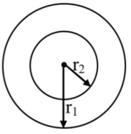Solution:

Electric field at a distance r,
(r0 < r < 2r0) from the center is given by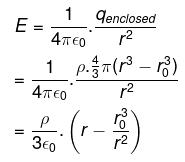Potential at the outer surface,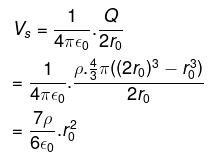∴ If V is the required potential at r  = 3r0/2,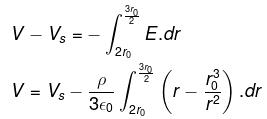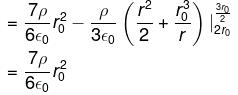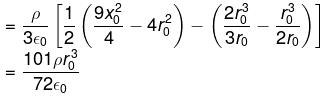QUESTION: 10

Electric field intensity at point ‘B’ due to a point charge ‘Q’ kept at a point ‘A’ is 12 NC-1 and the electric potential at a point ‘B’ due to same charge is 6 JC-1. The distance between AB is​

Solution:

E.l = V   where,
E = electric field intensity = 12 N/C
V = electric potential = 6 J/C
=> distance between A and B,
l = (6 / 12) m or (1 / 2) m = 0.5 m

QUESTION: 11

E = -dV/dr, here the negative sign signifies that​

Solution:

Solution :- The negative sign is just a convention and it signifies that the direction of E is opposite to the direction in which potential increases.

QUESTION: 12

Work done in carrying 2C charge in a circular path of radius 2m around a charge of 10C is​

Solution:

The overall work performed in carrying a 2coulomb charge in a circular orbit of radius 3 m around a charge of 10 coulomb is calculated below.
It is a well-known fact that W=qdv.
Here dV is the change in overall potential. In the circular orbit of r potential at each point is similar.
Most significantly, the value of r is 3.
The value of dv=0 and hence W=q0=0.

QUESTION: 13

Dimensional formula for potential difference is

Solution: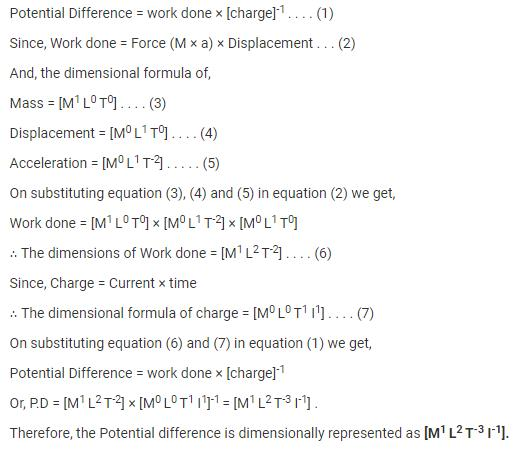QUESTION: 14

A point charge 2nC is located at origin. What is the potential at (1,0,0)?

Solution:

V = Q/(4πεr), where r = 1m
V = (2 X 10-9)/(4πε x 1) = 18 volts.

QUESTION: 15

If 100 J of work has to be done in moving an electric charge of 4C from a place where potential is -5 V to another place, where potential is V volt. The value of V is

Solution:

From the definition, the work done to a test charge ‘q0’ from one place to another place in an electric field is given by the formula
W=q0x[vfinal-vinitial ]
100=4x[v-(-5)]
v+5=25
v=20V

QUESTION: 16

A particle of charge q1 = 3μC is located on x-axis at the point x1 = 6 cm. A second point charge q2 = 2μC is placed on the x-axis at x2 = -4 cm. The absolute electric potential at the origin is​

Solution:

V=kq/r
Therefore, Vnet=Kx[(2µc/4) + (3µc/6)]
=9c109x10-6[1/2+1/2]x102
=9x105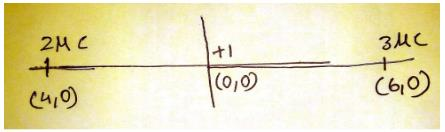QUESTION: 17

Electric potential is

Solution:

Electric potential is the electric potential energy per unit charge. In equation form, V=U/q, where U is the potential energy, q is the charge, and V is the electric potential. Since both the potential energy and charge are scalar quantities, so does the potential.
And it's dimension [ L2M1T-3I-1]

QUESTION: 18

A long, hollow conducting cylinder is kept coaxially inside another long, hollow conducting cylinder of larger radius. Both the cylinders are initially electrically neutral.

Solution:

When the charge is given to inner cylinder than there is an electric field is produced between cylinders which is given by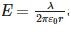and due to this a potential difference is developed between two cylinders.

QUESTION: 19

Equal charges are given to two spheres of different radii. The potential will

Solution:

When equal charges are given to two spheres of different radii, the potential will be more or the smaller sphere as per the equation, Potential = Charge / Radius.
Since potential is inversely proportional to radius, the smaller radius will have higher potential and vice versa.

QUESTION: 20

Consider a solid cube made up of insulating material having a uniform volume charge density. Assuming the electrostatic potential to be zero at infinity, the ratio of the potential at a corner of the cube to that at the centre will be

Solution: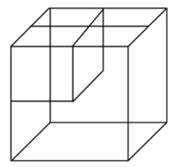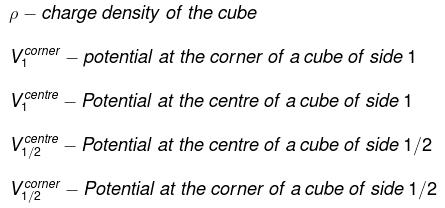By dimensional analysis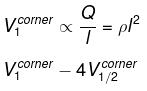but by superposition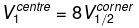Because of the centre of the larger cube lies at a corner of the eight smaller cubes of which it is made
therefore,